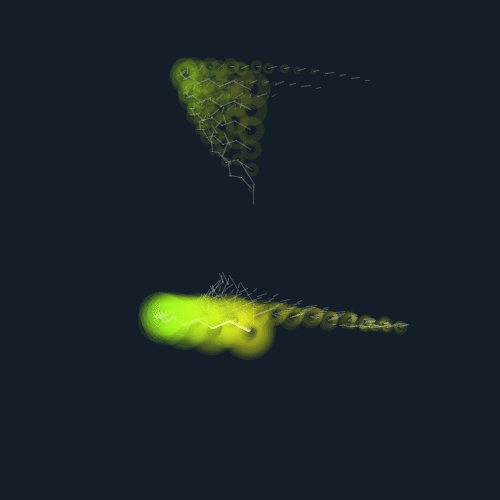# jackkoo

## 10 Feb 2015Click on it to see animation. A bunch of zigzags stacked together to create a living creature.This particle shape was sort of made by accident. I initially wanted it to pulse outwards like this.However I was playing with the array loop variables and notices that if I made their number of iteration increase through the array loop, they stack up and create a living looking creature. I decided I really like it and also wanted to show the structure. Therefore I made the top one as well so you can see each individual part, and then can imagine how the bottom one was made much easily.

```ArrayList<Tentacle> tentacles = new ArrayList<Tentacle>();
ArrayList<Zigzag> zigzags = new ArrayList<Zigzag>();

ArrayList<Tentacle> tentacles2 = new ArrayList<Tentacle>();
ArrayList<Zigzag> zigzags2 = new ArrayList<Zigzag>();

int numberOfTentacles = 20;
int numberOfTentacles2 = 8;

int frame = 0;

boolean a=false;

boolean b=false;
void setup()
{
for (int i = 0; i < numberOfTentacles; i++) {
Zigzag zigzag = new Zigzag
(
new Vec2(260, 250), // position
2 * i, // segments
new Vec2(10 +0.1 *i , 80 + 0.1 *i) // size
);

}

for (int i = 0; i < numberOfTentacles; i++){
Tentacle tentacle = new Tentacle(100, 100, zigzags.get(i).points);
}

for (int i = 0; i < numberOfTentacles2; i++) {
Zigzag zigzag = new Zigzag
(
new Vec2(260, 150 -(i*20)), // position
4 * i, // segments
new Vec2(10 , 80 ) // size
);

}

for (int i = 0; i < numberOfTentacles2; i++){
Tentacle tentacle = new Tentacle(100, 100, zigzags2.get(i).points);
}

size(500, 500);
frameRate(60);
}

void draw(){

background(20,30,40);
for (int i = 0; i < numberOfTentacles; i++){
tentacles.get(i).update();
}

for (int i = 0; i < numberOfTentacles2; i++){
tentacles2.get(i).update();
}

if(a){
if(b){
saveFrame("picture"+frame+".png");
frame++;
}
b=!b;
}
a = !a;

if(frame > 60){
noLoop();
}
}

class Joint{
Vec2 p1;
Vec2 p2;
Vec2 distance;

float woo;

float easeValue;
boolean easeDirection;

int numberOfJoints;
Joint nextJoint;

Joint (Vec2 p1, Vec2 p2, boolean easeDirection)
{
// create the center point
this.p1 = new Vec2(p1.x,p1.y);

// create the perimeter point, pointed up
this.p2 = new Vec2(p2.x, p2.y);

distance = new Vec2
(
abs(p1.x-p2.x),
abs(p1.y-p2.y)
);

this.easeValue = 0;
this.easeDirection = easeDirection;

woo = 2*PI/120;
}

void rotateJoint(float angle){
if(easeValue > 2*PI){
easeValue=0;
}

if(!easeDirection){
angle *= -1;
}
easeValue += woo;

float easeAngle = easeValue * angle;

//easeAngle = angle;
float s = sin(easeAngle);
float c = cos(easeAngle);

p2.x -= p1.x;
p2.y -= p1.y;

// rotate point
float xnew = distance.x * c - distance.y * s;
float ynew = distance.x * s + distance.y * c;

// translate point back:
p2.x = xnew + p1.x;
p2.y = ynew + p1.y;
}
}

class Tentacle {
float x;
float y;
ArrayList<Joint> joints = new ArrayList<Joint>();

Tentacle (float x, float y, ArrayList<Vec2> points){
this.x = x;
this.y = y;

boolean isLeft = false;

for (int i = 0; i < points.size()-1; i++){
Joint joint = new Joint
(
new Vec2(points.get(i+1).x, points.get(i+1).y),
new Vec2(points.get(i).x , points.get(i).y),
isLeft
);

isLeft = !isLeft;
}
}

void update(){

for(int i = 0; i < joints.size()-1; i++){
Joint joint = joints.get(i+1);
Joint jointNext = joints.get(i);
noFill();

jointNext.p1.x = joint.p2.x;
jointNext.p1.y = joint.p2.y;

strokeWeight(1);
stroke(255,255,255,60);
line
(
joint.p1.x,
joint.p1.y,
joint.p2.x,
joint.p2.y
);

strokeWeight(i/2);
stroke(joint.p1.x, joint.p1.x*i, 30, 20);
float ellipseSize = i/1 * 1.1;
ellipse(joint.p1.x, joint.p1.y, ellipseSize, ellipseSize);
joint.rotateJoint(1);

//ellipse(joint.p2.x, joint.p2.y, 10,10);
}
}
}

class Vec2{
float x;
float y;

Vec2 (float x, float y)
{
this.x = x;
this.y = y;
}
}

class Zigzag{
ArrayList<Vec2> points = new ArrayList<Vec2>();

Zigzag(Vec2 position, int numberOfSegments, Vec2 segmentSize){
// Create a uniform zigzag
for (int i = 0; i < numberOfSegments; i++){
Vec2 vec2 = new Vec2(position.x, position.y + segmentSize.y * PennerEaseOutSine((float)i/numberOfSegments));

// Change zigzag direction

}

// Create the zigzag
for (int i = 0; i < points.size(); i++){
points.get(i).x += segmentSize.x * PennerEaseInSine((float)i/numberOfSegments);
segmentSize.x*=-1;
}
}

void render(){
noFill();
beginShape();

for (int i = 0; i < points.size(); i++){
strokeWeight(i/4);
stroke(i*25,i*35,0);
float ellipseSize = i/1 * 1.1;
ellipse(points.get(i).x, points.get(i).y, ellipseSize, ellipseSize);

stroke(90,90,0);
strokeWeight(0.4);
vertex(points.get(i).x, points.get(i).y );
}

endShape();
}
}```